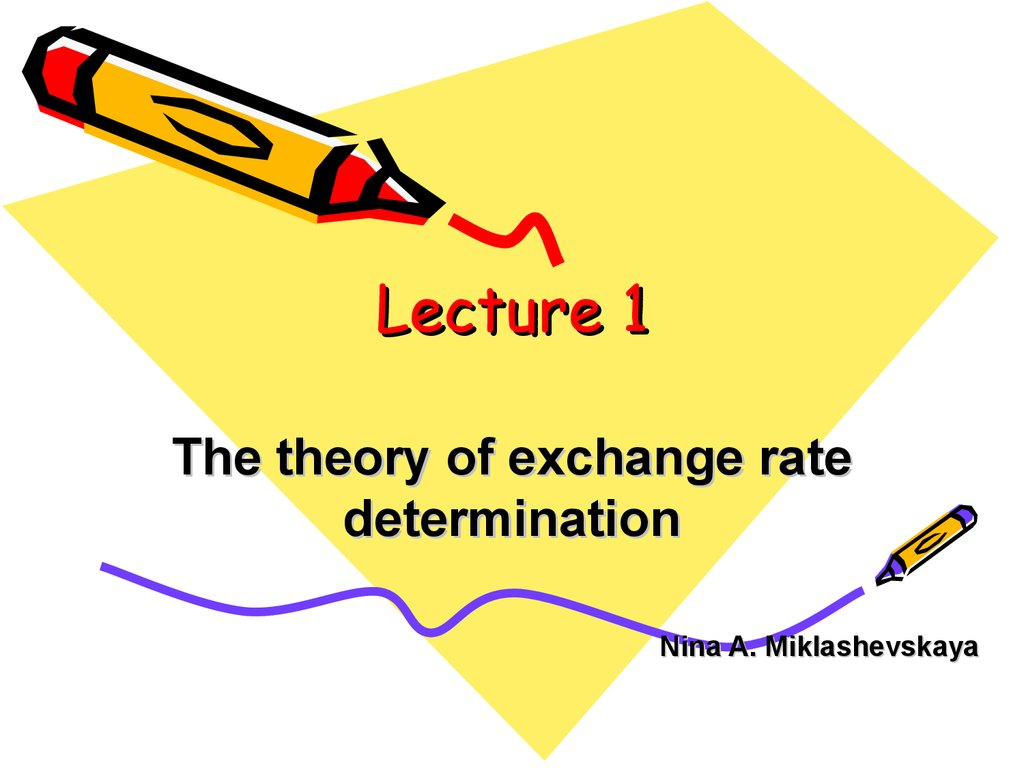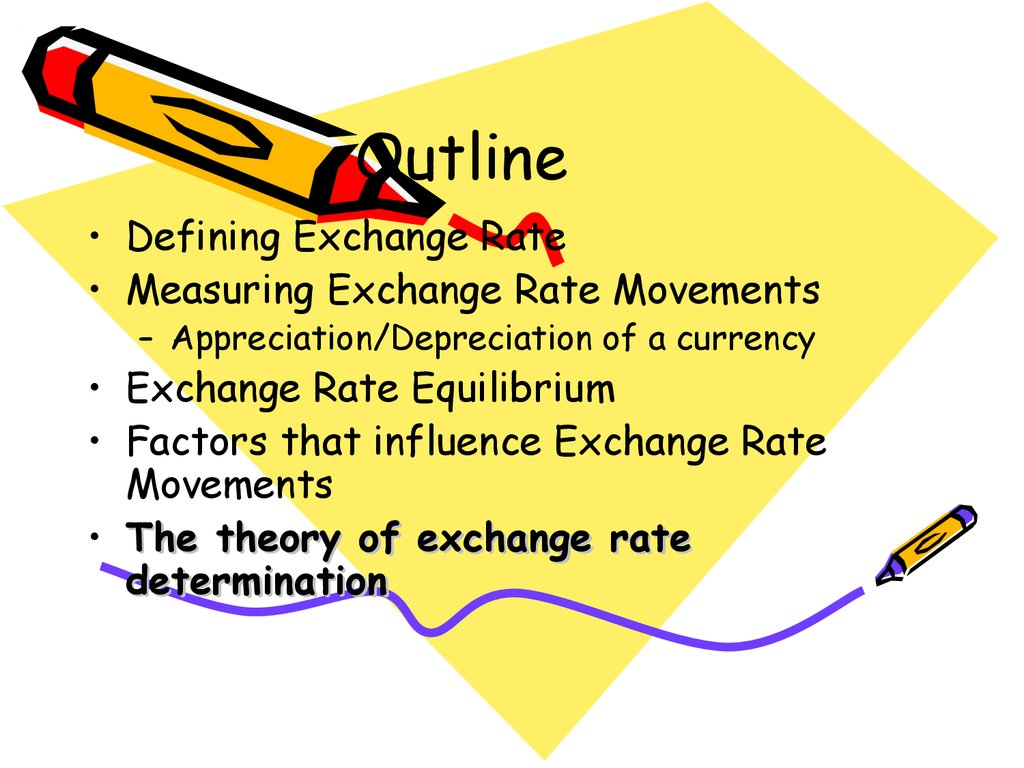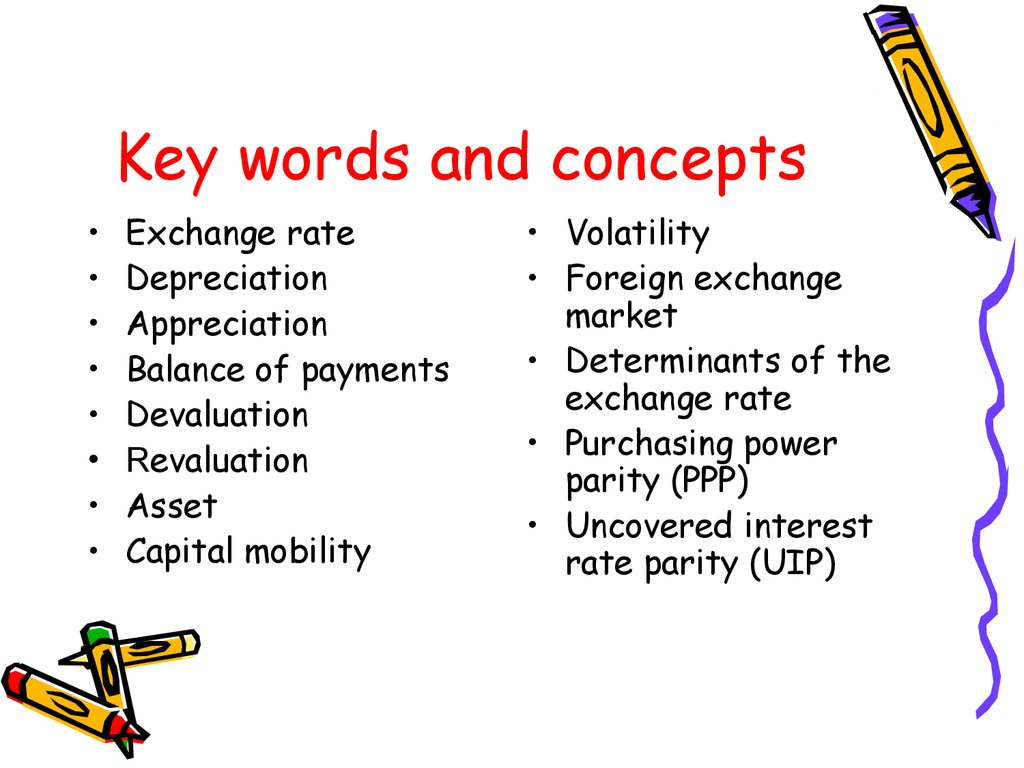# The theory of exchange rate determination

## 1. Lecture 1

The theory of exchange rate
determination
Nina A. Miklashevskaya

## 2. Outline

• Defining Exchange Rate
• Measuring Exchange Rate Movements
– Appreciation/Depreciation of a currency
• Exchange Rate Equilibrium
• Factors that influence Exchange Rate
Movements
• The theory of exchange rate
determination

## 3. Key words and concepts

Exchange rate
Depreciation
Appreciation
Balance of payments
Devaluation
Revaluation
Asset
Capital mobility
• Volatility
• Foreign exchange
market
• Determinants of the
exchange rate
parity (PPP)
• Uncovered interest
rate parity (UIP)

## 4. What does it mean EXCHANGE RATE?

Nominal exchange rate
Spot rate
Forward rate
Bilateral exchange rate
Effective exchange rate
Real exchange rate

## 5. Meaning of Nominal Exchange Rate

• Nominal exchange rate is the relative price of the currency
of two countries or value of one currency in units of another
currency
• An exchange rate can be quoted in two ways:
– Direct
• The price of the foreign currency in terms of domestic
currency (the number of rubles needed to purchase
one U.S. dollar)
– Indirect
• The price of domestic currency (pound) in terms of the
foreign currency

## 6. Measuring Changes in Exchange Rates

• A decline in a local currency’s value is
referred to as depreciation and an
increase in local currency’s value is called
appreciation.
• If foreign currency A can buy you more units of
local currency, currency A has appreciated and
local currency depreciated
• If foreign currency A can buy you less units of
local currency, currency A has depreciated and
local currency appreciated

## 7. Appreciation/Depreciation

• Percentage change in value of Foreign
Currency
New Value of one \$ in terms of
local Currency
- Old value of one \$ in terms of local currency
-------------------------------------------------- X 100
Old value of one \$ in terms of local Currency
• Depreciation of home country’s currency makes home goods cheaper for
foreigners and foreign goods more expensive for domestic residents.
• Appreciation of home country’s currency makes home goods more
expensive for foreigners and foreign goods cheaper for domestic
residents.

## 8. Exchange Rates and International Transactions

• Exchange Rates and Relative Prices
– Import and export demands are influenced
by relative prices.
– Appreciation of a country’s currency:
• Raises the relative price of its exports
• Lowers the relative price of its imports
– Depreciation of a country’s currency:
• Lowers the relative price of its exports
• Raises the relative price of its imports

## 9. Nominal Exchange Rate

• Bilateral exchange rate is the rate at
which you can swap the money of one
country for that of another
• Nominal effective exchange rate is a
measure of how the local currency does on
average against all countries currencies
• EE=(E\$)α(E€)β(E¥)γ α+β+γ=1

## 10. The importance of exchange rate

Price unification of goods produced in different countries - they
enable us to translate different counties’ prices into comparable
terms
Influence on the competitiveness of domestic goods in the foreign
markets
Impact on exports and imports. If we know the exchange rate
between two countries’ currencies, we can compute the price of one
country’s exports in terms of the other country’s money.
• Example: The dollar price of a £50 sweater with a dollar exchange rate of
\$1.50 per pound is (1.50 \$/£) x (£50) = \$75.
Influence on the relative price of assets and the level of capital
mobility
Affect macroeconomic stability, the inflation rate and inflationary
expectations

## 11. The Foreign Exchange Market

• Exchange rates are determined in the foreign
exchange market.
– The market in which international currency trades take place
• The Actors
– The major participants in the foreign exchange market are:
• Commercial banks
• International corporations
• Nonbank financial institutions
• Central banks
- Foreign currency trading among banks
• It accounts for most of the activity in the foreign exchange
market

## 12.

Functions of FX Market
The foreign exchange market is the
mechanism by which participants:
– transfer purchasing power across countries;
– obtain or provide credit for international
– minimize exposure to the risks of exchange
rate changes
12

## 13. Exchange Rates and International Transactions

• Spot Rates and Forward Rates
– Spot exchange rates
• Apply to exchange currencies “on the spot”
– Forward exchange rates
• Apply to exchange currencies on some future
date at a prenegotiated exchange rate
– Forward and spot exchange rates, while not
necessarily equal, do move closely together.

## 14. Exchange Rate Equilibrium

• Forces of Demand and Supply
• Demand for foreign currency negatively
related to the price of foreign currency
• Supply of foreign currency positively
related to the price of foreign currency
• Forces of demand and supply together
determine the exchange rate

## 15. Foreign Exchange Market

• At the equilibrium exchange rate Е* ,
the demand for foreign currency
equals the supply of foreign currency
E Rub./\$
E*¹
E*º

D\$

S\$ An increase in the demand
for \$\$ causes a
depreciation of the ruble
D\$¹
\$\$

## 16. Exchange rate regimes

• Floating exchange rates – the CB allows the
currency to depreciate until the balance of
payments deficit is eliminated
• (the exchange rate as automatic mechanism of
• Fixed exchange rates – the CB intervenes in the
foreign exchange market functioning. It loses
foreign exchange reserves in case of a balance of
payments deficit.
• Devaluation/revaluation of domestic currency

## 17. Real Exchange Rate

• The real exchange rate (RER) is the
relative price of the goods of two
countries.
• RER is the rate at which we can
trade the goods of one country for
the goods of another

## 18. Real Exchange Rate

EP *
R
P
E – nominal exchange rate
Р* /P – ratio of price levels
• Real effective exchange rate –
• REER=(E\$ P\$*/P)α(E€P€/P)β(E¥P¥/P)γ α+β+γ=1
• Real depreciation results an increase in net exports
(NX)

## 19. Real Exchange Rate

EPT *
R
PN
E – nominal exchange rate
РT* - prices of tradables in foreign currency
РN - prices of nontradables in local currency
• Real depreciation results an increase in net exports

## 20. Real Exchange Rate

EW *
R
W
E - nominal exchange rate
W* - unit labor costs abroad (in foreign currency)
W - unit labor costs in home country (in local currency)
• Real depreciation results an increase in net exports

## 21. Real Exchange Rate

EPIM *
R
PEX
E - nominal exchange rate
РIM* - prices of importable goods in foreign currency
РEX – prices of exportable goods in local currency
• Real depreciation results an increase in net exports

## 22. Why the RER matters

• Real variable
• RER determines the allocation of
resources
• Impact on the competitiveness

## 23. Current Account Theories

• The law of one price - the same good can not sell
for different prices in different locations at the
same time
exchange rate will
• Hypothesis that the nominal
e
will be the same in every country
EP *
R
1
P
P
e
P*

## 25. PPP Model as Special Case

• PPP model is a special case of the real exchange
rate
– Implies that real exchange rate is fixed at unity
• No change in real exchange rate
– However real exchange rates do change therefore there must
be important elements of the real world that the PPP theory
ignores
» PPP assumes all goods entering into the price levels of both
» Tariffs and transaction costs
» Phenomenon of product differentiation
» Allows for separate markets (and therefore prices) for
import and domestic varieties of a good

## 26. PPP Model as Special Case

• Real exchange rate equation captures
reality at any point in time
– PPP relationship never holds exactly
• PPP equation gives a sense of a long-term
tendency towards which nominal exchange
rates move absent other changes

## 27. Exchange Rates in the LR

• PPP holds
• Relative prices are constant.
Therefore, the real exchange rate
equals one
• The nominal exchange rate returns to
its “fundamentals”

## 28.

Monetary Approach to exchange rates and the
“fundamentals” for a currency
Foreign Money Market
Domestic Money Market
M
P 1 i
y
*
M
P* 1 i* *
y
PPP
P eP
*
*
M Y 1 i
e *
*
M Y 1 i
This should give us the long run trend

## 29. Exchange Rates in the SR

• Commodity prices are fixed (PPP
fails)
• UIP and Currency markets determine
exchange rates

## 31. The Demand for Foreign Currency Assets

• The demand for a foreign currency bank
deposit is influenced by the same
considerations that influence the demand
for any other asset.
• Assets and Asset Returns
– Defining Asset Returns
• The percentage increase in value an asset offers
over some time period.
– The Real Rate of Return
• The rate of return computed by measuring asset
of products that savers regularly purchase.

## 32. The Demand for Foreign Currency Assets

• Risk and Liquidity
– Savers care about two main characteristics
of an asset other than its return:
• Risk
– The variability it contributes to savers’ wealth
• Liquidity
– The ease with which it can be sold or exchanged for
goods

## 33. The Demand for Foreign Currency Assets

• Return, Risk, and Liquidity in the
Foreign Exchange Market
– The demand for foreign currency assets depends not only on
returns but on risk and liquidity.
• There is no consensus among economists about the importance
of risk in the foreign exchange market.
• Most of the market participants that are influenced by liquidity
factors are involved in international trade.
– Payments connected with international trade make up a very
small fraction of total foreign exchange transactions.
– Therefore, we ignore the risk and liquidity motives for holding
foreign currencies (perfect capital mobility)

## 34. The Demand for Foreign Currency Assets

• Interest Rates
– Market participants need two pieces of
information in order to compare returns on
different deposits:
• How the money values of the deposits will change
• How exchange rates will change
– A currency’s interest rate is the amount of
that currency an individual can earn by
lending a unit of the currency for a year.
• Example: At a dollar interest rate of 10% per year, the
lender of \$1 receives \$1.10 at the end of the year.

## 35.

The Demand for
Foreign Currency Assets
• Exchange Rates and Asset Returns
– The returns on deposits traded in the
foreign exchange market depend on
interest rates and expected exchange rate
changes.
– In order to decide whether to buy a euro
or a dollar deposit, one must calculate the
dollar return on a euro deposit.

## 36.

The Demand for
Foreign Currency Assets
• A Simple Rule
– The dollar rate of return on euro deposits
is approximately the euro interest rate
plus the rate of depreciation of the
dollar against the euro.
• The rate of depreciation of the dollar
against the euro is the percentage increase in
the dollar/euro exchange rate over a year.

## 37. The Demand for Foreign Currency Assets

– The expected rate of return difference between dollar and euro
deposits is:
R\$ = R€ + (Ee\$/ € - E\$/€ )/E\$/€
(1)
where:
R\$
= interest rate on one-year dollar deposits
R€ = today’s interest rate on one-year euro deposits
E\$/€
= today’s dollar/euro exchange rate (number of
dollars per euro)
Ee\$/€
= dollar/euro exchange rate (number of dollars per
euro) expected to prevail a year from today

## 38. Uncovered Interest Rate Parity (UIP)

• A parity condition stating that the difference in
interest rates between two countries is equal to
the expected change in exchange rates between
the countries’ currencies. If this parity does not
exist, there is an opportunity to make a profit.
"i1" represents the interest rate of country 1
"i2" represents the interest rate of country 2
"E(e)" represents the expected rate of change in the exchange rate

## 39. Equilibrium in the Foreign Exchange Market

• Interest Parity: The Basic Equilibrium
Condition
– The foreign exchange market is in equilibrium when deposits
of all currencies offer the same expected rate of return.
– Interest parity condition
• The expected returns on deposits of any two currencies
are equal when measured in the same currency.
• It implies that potential holders of foreign currency
deposits view them all as equally desirable assets.
• The expected rates of return are equal when:
R\$ = R€ + (Ee\$/€ - E\$/€)/E\$/€
(2)

## 40. Equilibrium in the Foreign Exchange Market

• How Changes in the Current Exchange
Rate Affect Expected Returns
– Depreciation of a country’s currency today
lowers the expected domestic currency
return on foreign currency deposits.
– Appreciation of the domestic currency
today raises the domestic currency return
expected of foreign currency deposits.

## 41.

Equilibrium in the
Foreign Exchange Market
Today’s Dollar/Euro Exchange Rate and the Expected Dollar
Return on Euro Deposits When Ee\$/€ = \$1.05 per Euro

## 42.

Equilibrium in the
Foreign Exchange Market
The Relation Between the Current Dollar/Euro Exchange Rate
and the Expected Dollar Return on Euro Deposits
Today’s dollar/euro
exchange rate, E\$/€
1.07
1.05
1.03
1.02
1.00
0.031
0.050 0.069 0.079 0.100
Expected dollar return on
euro deposits, R€ + (Ee\$/€ - E\$/€)/
(E\$/€)

## 43. Equilibrium in the Foreign Exchange Market

• The Equilibrium Exchange Rate
– Exchange rates always adjust to
maintain interest parity.
– Assume that the dollar interest rate R\$,
the euro interest rate R€, and the
expected future dollar/euro exchange
rate Ee\$/€, are all given.

## 44. Equilibrium in the Foreign Exchange Market

Determination of the Equilibrium Dollar/Euro Exchange Rate
Exchange rate, E\$/€
E2\$/€
E1\$/€
E
Return on
dollar deposits
2
1
3
3
\$/€
Expected return
on euro deposits
R\$
Rates of return
(in dollar terms)

## 45. Interest Rates, Expectations, and Equilibrium

• The Effect of Changing Interest Rates on
the Current Exchange Rate
– An increase in the interest rate paid on
deposits of a currency causes that currency
to appreciate against foreign currencies.
• A rise in dollar interest rates causes the dollar to
appreciate against the euro.
• A rise in euro interest rates causes the dollar to
depreciate against the euro.

## 46. Interest Rates, Expectations, and Equilibrium

Effect of a Rise in the Dollar Interest Rate
Exchange rate, E\$/€
E1\$/€
Dollar return
1
E2\$/€
1'
2
Expected
euro return
R1\$
R2\$
Rates of return
(in dollar terms)

## 47. Interest Rates, Expectations, and Equilibrium

Effect of a Rise in the Euro Interest Rate
Exchange rate, E\$/€
Dollar return
Rise in euro
interest rate
E2\$/€
2
E1\$/€
1
Expected
euro return
R\$
Rates of return
(in dollar terms)

## 48. Interest Rates, Expectations, and Equilibrium

• The Effect of Changing Expectations
on the Current Exchange Rate
– A rise in the expected future exchange
rate causes a rise in the current exchange
rate.
– A fall in the expected future exchange
rate causes a fall in the current exchange
rate.

## 49. Factors that influence the Exchange Rate

• Expectations of the Market
• Political Events
• Relative Inflation Rates
• Relative Interest Rates
• Relative Income Levels
Exchange rate is the results of an
interaction of these factors

## 50. Outcomes

• Models of exchange rate
determination based on
macroeconomic fundamentals have not
had much success in either explaining
or forecasting exchange rates,
possibly owing to the simplifying
assumptions employed.

## 51. Micro-based models of exchange rates

•Information is dispersed
•Investors are heterogeneous